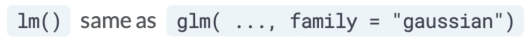Tutorials
r programming

# GLM in R: Generalized Linear Model

Generalized linear model (GLM) is a generalization of ordinary linear regression that allows for response variables that have error distribution models other than a normal distribution like Gaussian distribution.

## Basics of GLM

GLMs are fit with function glm(). Like linear models (lm()s), glm()s have formulas and data as inputs, but also have a family input.

### Generalized Linear Model SyntaxThe Gaussian family is how R refers to the normal distribution and is the default for a glm().

### Similarity to Linear Models

If the family is Gaussian then a GLM is the same as an LM.### Non-normal errors or distributions

Generalized linear models can have non-normal errors or distributions. However, there are limitations to the possible distributions. For example, you can use Poisson family for count data, or you can use binomial family for binomial data.

GLMs also have a non-linear link functions, which links the regression coefficients to the distribution and allows the linear model to generalize.

## Interactive Example of Predicting with glm()

This example predicts the expected number of daily civilian fire injury victims for the North American summer months of June, July, and August using the Poisson regression you and the newDat dataset.

Here is the data in the newDat dataset:

  Month
1     6
2     7
3     8


The Poisson slope and intercept estimates are on the natural log scale and can be exponentiated to be more easily understood. You can do this by specifying type = "response" with the predict function.

# use the model to predict with new data
predOut <- predict(object = poissonOut, newdata = newDat, type = "response")

# print the predictions
print(predOut)


When we run the above code, it produces the following result:

         1          2          3
0.08611111 0.12365591 0.07795699